College Physics : Universal Gravitation

Example Questions

Example Question #1 : Forces

Two small lead balls of masses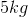and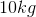are fixed a distance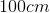apart on a laboratory bench. A student moves the larger mass toward the smaller mass so the distance is now only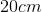. After the mass was moved, by what factor did the gravitational force between the lead balls change?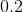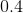Explanation:

The gravitational force obeys an inverse-square law. Decreasing the distance by a factor ofwill increase the force by a factor of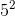, or.

Example Question #55 : College Physics

Which of these equations is the universal gravitational equation?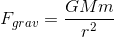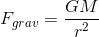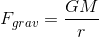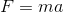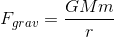Explanation:is the equation for Universal gravitation.

Example Question #1 : Universal Gravitation

The gravitational force between two objects is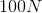. What would be the force of gravity between them if the mass of one of the objects is tripled and the distance between them is doubled?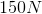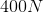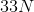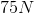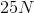Explanation:

So the fact that we don't actually know any of the numbers doesn't matter.

What matters is we have the equation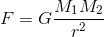The masses have a direct proportional relationship with the force. That means that if a mass doubles, the force doubles.

We can also see that the radius (distance between the masses) has an inverse square relationship with Force. So if we double the radius, we divide the force by 4.

So based on the problem, we triple a mass and double the radius. So we triple the force then divide by 4.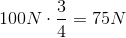All College Physics Resources